Courses

# Soil Mechanics - 5

## 10 Questions MCQ Test GATE Civil Engineering (CE) 2022 Mock Test Series | Soil Mechanics - 5

Description
This mock test of Soil Mechanics - 5 for Civil Engineering (CE) helps you for every Civil Engineering (CE) entrance exam. This contains 10 Multiple Choice Questions for Civil Engineering (CE) Soil Mechanics - 5 (mcq) to study with solutions a complete question bank. The solved questions answers in this Soil Mechanics - 5 quiz give you a good mix of easy questions and tough questions. Civil Engineering (CE) students definitely take this Soil Mechanics - 5 exercise for a better result in the exam. You can find other Soil Mechanics - 5 extra questions, long questions & short questions for Civil Engineering (CE) on EduRev as well by searching above.
QUESTION: 1

### Which of the following is NOT an assumption in Terzaghi’s one-dimensional consolidation theory?

Solution:

Assumptions in Terzaghi’s theory of consolidation:

1. The soil is homogeneous.

2. The soil is fully saturated.

3. The compression of the soil layer is due to the change in volume only, which, in turn, is due to the squeezing out of water from the void spaces.

4. Darcy’s law is valid.

5. Deformation of soil occurs only in the direction of the load application.

6. The coefficient of consolidation is constant during the consolidation.

QUESTION: 2

### Match List – I (Equipment) with List-II (Purpose) and select the correct answer using the codes given below the lists: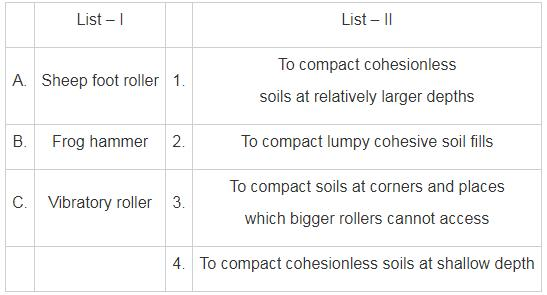Solution: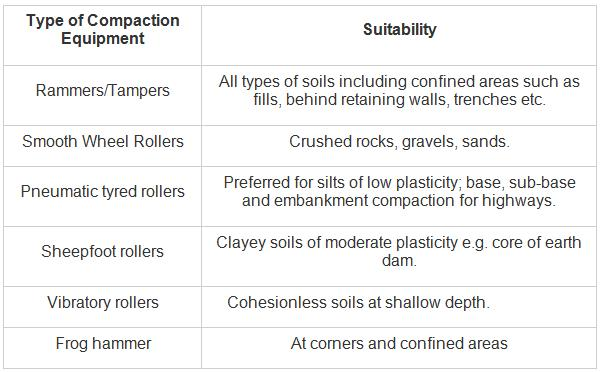QUESTION: 3

### Choose the correct statement 1. Soils with small volume changes are compacted to the dry side of optimum. 2. Soils with large volume changes are compacted to the wet side of optimum.

Solution: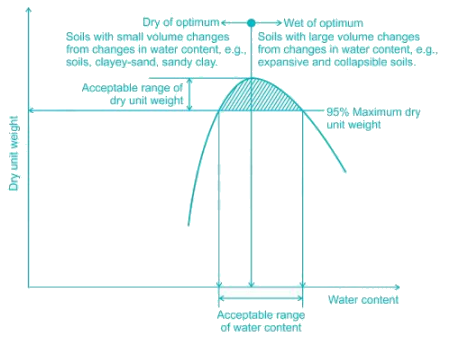QUESTION: 4

Time factor for 90% consolidation is 0.848

Time factor for 50% consolidation is 0.197

The time required for 90% consolidation is 30 Mins

The time required for 50% consolidation is 9 Mins

Length of drainage path is 10mm

The Coefficient of consolidation of the sample by the square root of time fitting method is

Solution:

Cv = (T90 x d2)/ t90 = 0.848 x 0.012/ (30x60) =  4.71 x 10-8 m2/s

QUESTION: 5

Consider the following statements :

1. In the laboratory consolidation test, initial compression is the result of the displacement of soil particles.

2. Primary consolidation is due to dissipation of pore water pressure.

3. Secondary compression starts after complete dissipation of pore water pressure.

4. Primary consolidation and secondary compression occur simultaneously.

Which of the statements given above are correct?

Solution:

In laboratory consolidation test, the soil is laterally confined and fully saturated. So there is almost no opportunity for particles to get displaced and cause initial compression. Primary consolidation is due to dissipation of pore water pressure. The time taken to dissipate excess pore pressure and transfer of stress increment in this stage is called hydrodynamic lag. After this stage is complete, the stresses are transferred to the surface of the soil particles.

It should be noted that secondary compression starts after the completion of primary consolidation.

QUESTION: 6

Consider the following statements

P. For a given compactive effort, the permeability decreases sharply with the increase in water content on the dry side of optimum.

Q. For a given compactive effort, the soil tends to have a dispersed structure on the dry of optimum

R. For a given compactive effort, the soil tends to have a flocculated structure on the wet of optimum

S. Soils compacted on the dry of optimum tend to exhibit more shrinkage upon drying than those compacted wet of optimum

Solution:

Effect of compaction on structure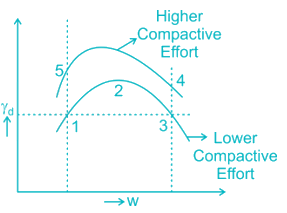At point 1:Flocculent structure is found on dry side of optimum and with increase in water content of soil there will be decrease in permeability of the soil due to improved orientation of the particles which results in a decrease in the size of voids.

At point 3:At same compactive effort dispersed structure is found on wet side of optimum

Effect of shrinkage: Due to random orientation of the soil particles and stronger inter-particle bonds, shrinkage on dry of optimum side is found to be less than shrinkage on wet of optimum side.

Compactive effort: With increase in comp active effort at same water content the soil tend to become more oriented (dispersed) especially on the dry side of optimum.

Point 5:QUESTION: 7

The compaction of on embankment is carried out in 300 mm thickness of lifts. The rammer used has foot area of 0.05 sq. m. The energy developed per drop of the rammer is 40 kg-m. Assuming 50% more energy in each pas over the compacted layer due to overlap, calculate a number of purses required to develop compaction energy equivalent to IS light compaction for each layer.

Solution:

Energy in standard proctor test

= (Wt. of hammer (kg) × free fall (m) × layer × blows per layer)/ (volume of soil mass taken)

Volume of soil mass taken = 1000 cc ≈ 1 lit.

Energy in standard proctor test = (2.6 × 0.310 × 3 × 25)/10-3

= 60450 kg m/m3

60450 = [40/(0.3 × 0.05)] × 1.5 × n             (50% due to overlap)

n → no. of passes

∴ n = 15.11 ≈ 15

QUESTION: 8

To achieve 80% consolidation in a clay layer, the time required will be 15 years. If the clay layer is twice as thick, 15 times more permeable and 10 times less compressible, then the time that would be required to achieve the same degree of consolidation will be

Solution:

Concept:

Using the relation,

Time factor (TV) is given by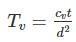Where t = time required to consolidate the clay layer by (U%).

d = thickness of clay layer

cv = coefficient of consolidation

Also,

K = cv mv γw

Where,

K = permeability of soil sample

cv = coefficient of consolidation

mv = coefficient of volume compressibility

Calculation:

For U = 80%,
TV = TV1 and time required will be 15 years.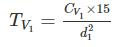Now for same U, TV = TV2 = TV1 but d2 = 2d1, k2 = 15k1, mv2 = 110mv1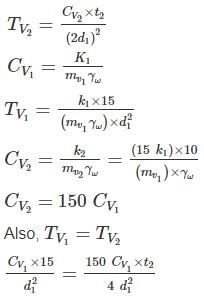t2 = 0.4 years

t2 = 4.8 months

*Answer can only contain numeric values
QUESTION: 9

A soil sample is tested in a consolidometer shows compression of 0.75 mm when the load is increased from 50 kN/m2 to 100 kN/m2. If instead of 100 kN/m2, the load is increased to 150 kN/m2, settlement of the soil sample will be _____ mm.

Solution:

Consolidation settlement is given by: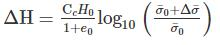Where,

CC = Compression index

e0 = Initial void ratio of the soil sample

H0 = Thickness of the soil sample

σ̅0 = Initial effective stress at the centre of the soil sample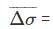Increase in effective stress at the centre of the soil sample

Calculation:

ΔH1 = 0.75 mm

σ̅0 = 50 kN/m2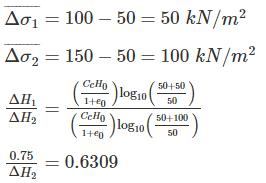ΔH2 = 1.188 mm

*Answer can only contain numeric values
QUESTION: 10

A rectangular footing (assume it to be rigid) 3m × 5m in plan rests on a cohesive soil having Modulus of elasticity 5.5 × 104 kN/m2 and Poisson's ratio 0.45. If the immediate settlement at the corner of the footing is not to exceed 4.3mm, then calculate the safe load (kN) that can be applied at the base of the footing against the Factor of Safety of 1.5.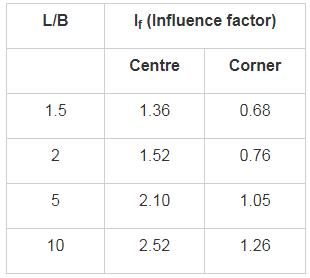Solution:

Immediate settlement in cohesive soil/clay is given by:

q = uniform pressure at the base of foundation

B = width of the footing

E = Modulus of Elasticity of soil

μ = poisson’s ratio

If = Influence factor which depend upon L/B ratio

Calculation:

L = 5 m

B = 3 m
L/B = 5/3 = 1.67
From the table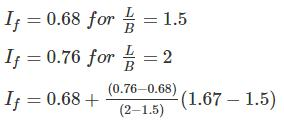If = 0.707

Maximum permissible settlement = 4.3 mm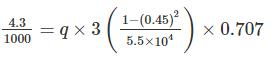q = 139.82 kN/m2

Safe pressure at Base of foundation = 139.82/FOS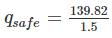qsafe = 93.21 kN/m2

Safe load = 93.21 × 3 × 5 = 1398.17 kN

Safe load = 1398.17 kN